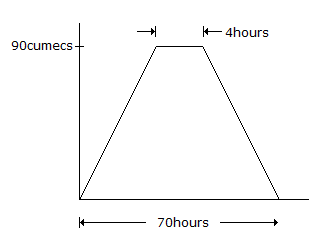# Civil Engineering - GATE Exam Questions - Discussion

### Discussion :: GATE Exam Questions - Section 4 (Q.No.12)

12.

A direct runoff hydrograph due to an isolated storm with an effective rainfall of 2 cm was trapezoidal in shape as shown in the below figure. The hydrograph corresponds to a catchment area (in sq. km) of[A]. 790.2 [B]. 599.4 [C]. 689.5 [D]. 435.3

Explanation:

No answer description available for this question.

 Pulkit said: (Dec 16, 2016) AREA = 90 * 1/2 * (70 + 4) * 60 * 60 * 10^(-6). 60 * 60 to convert hr to secs, 10^(-6) for sqm to sq km.

 Rakesh Kumar said: (Jan 30, 2017) Can anyone explain even more clearly?

 Akhil said: (Jun 25, 2017) Area =1/2 * sum of parallel side *height. = 1/2*(70+4) * 900 *60*60*(10)^(-6), 60*60 is conversion hour to secs 10^(-6) sqm to sqkm.

 Sarvesh said: (Feb 14, 2018) 1/2*(70+4)*900*60*60*(10)^(-6) = 199.88 * 5 = 599.4.

 Reshma said: (Apr 15, 2018) Why 900, is it 90 cumecs right?

 Keshav Kaushik said: (Jan 9, 2019) How come 900 and why 5 has been used?

 Yesika said: (Jun 21, 2019) Area of given DRH = volume of direct run off. [(1/2)(70+4) * (60)*(60) * (90) ] m3=11988000 m3, Effective rainfall = 2 cm, Catchment area = Volume of DRH/Effective rainfall. 11988000 / (2 * 10-2) m2 = 599.4 m2.